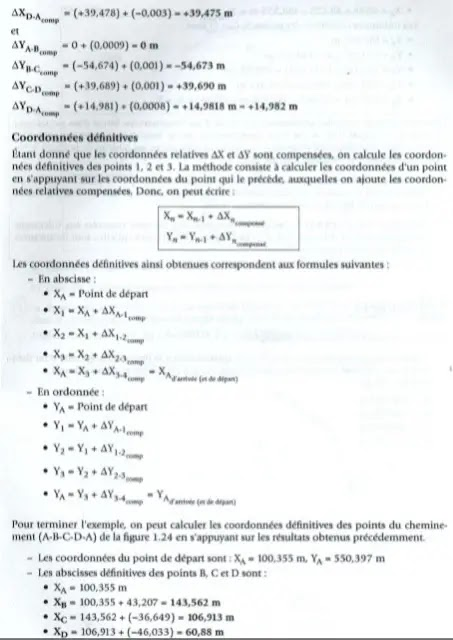Definition of Polygonation Types of Traverse

# Definition of Polygonation Types of Traverse### Definition of Polygonation  and Calculation  of Topographic Traverse Types

☼ POLYGONATION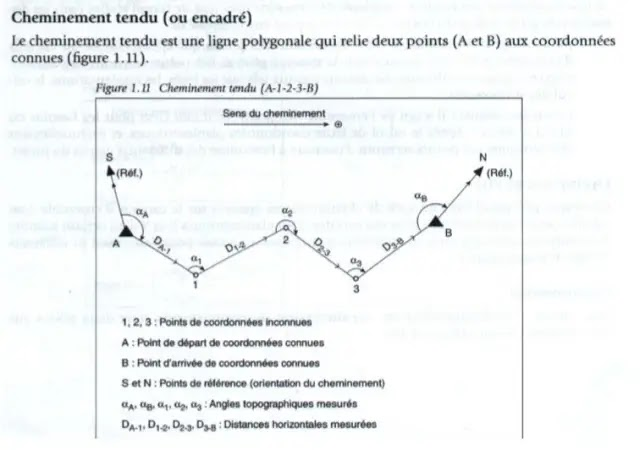₪ 2. Closed path₪ 3. Calculation of a tense path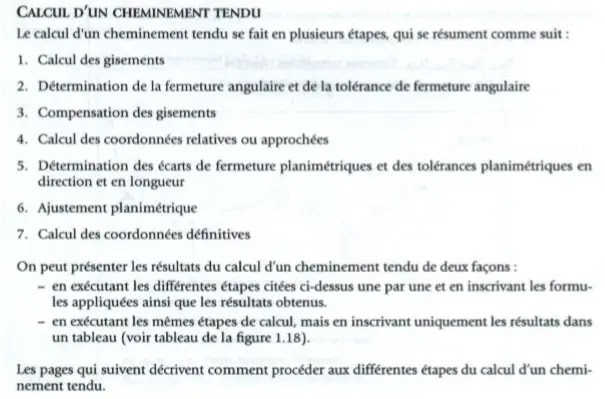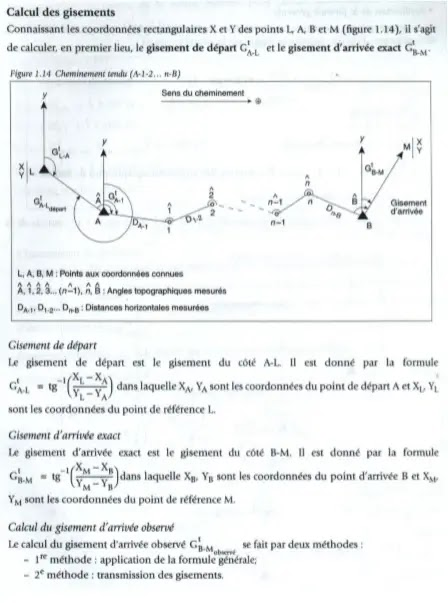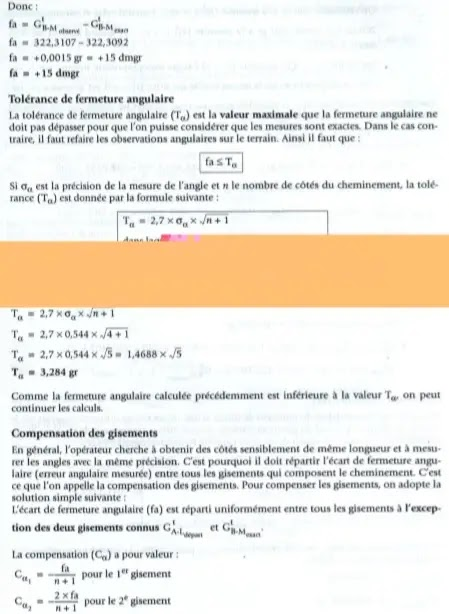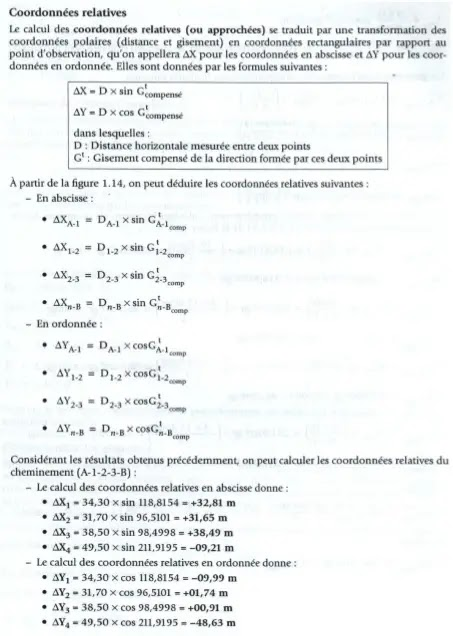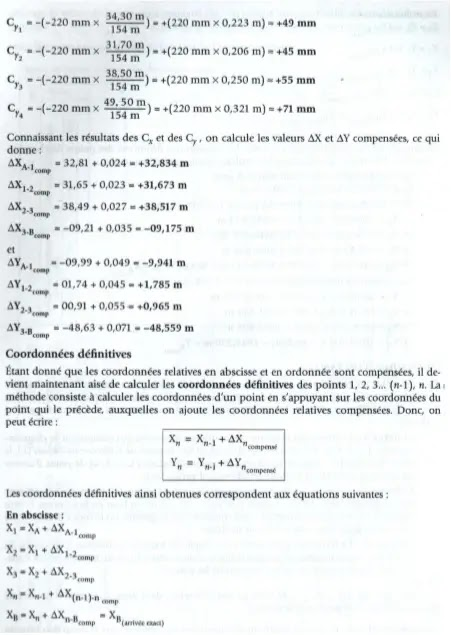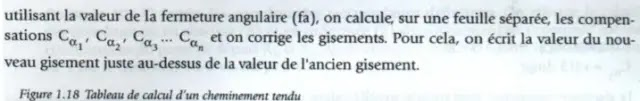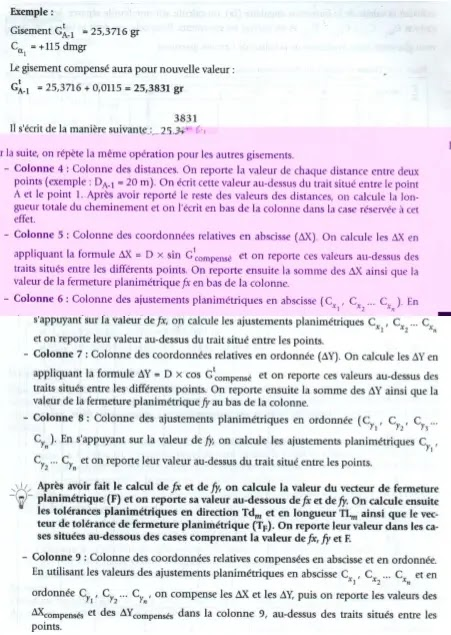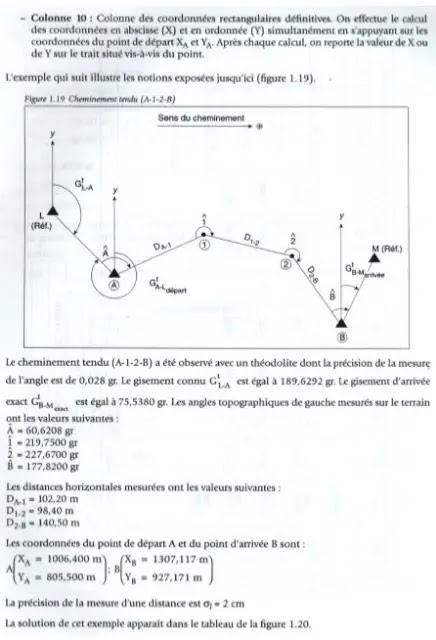₪ 4. Calculation of a closed path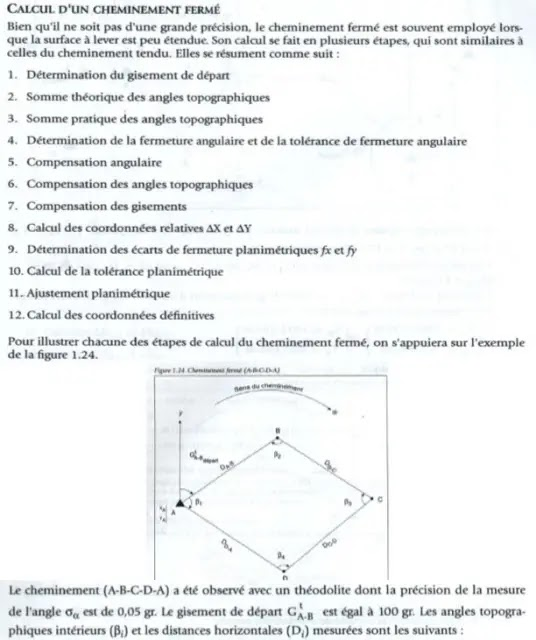₪ β 1 = 176.9400 g
β2 = 62,4400gr
β3 = 92,3200gr
β4 = 68,4200gr
DA-B = 43,210 m
DB-= 65,818 m
D C - D = 60.778 m
DD - A = 42.225 m
The coordinates of the starting point   A  are:
XA =  100.355m ;  YA = 550.397m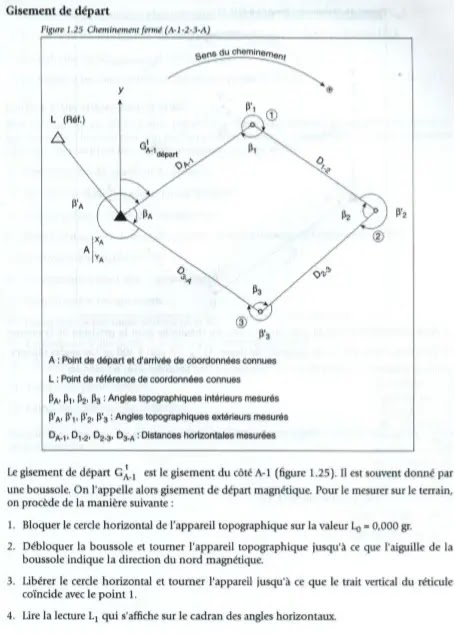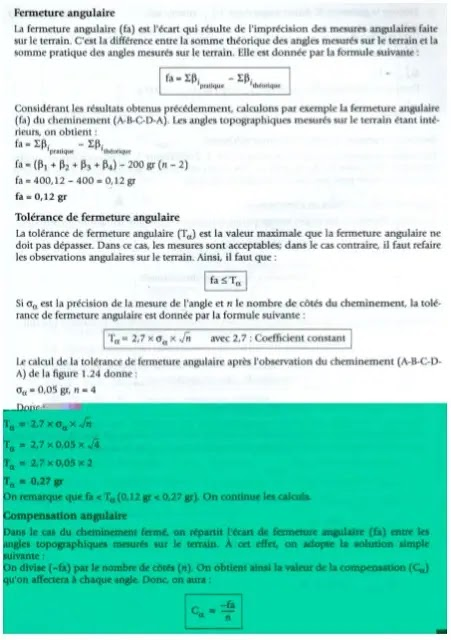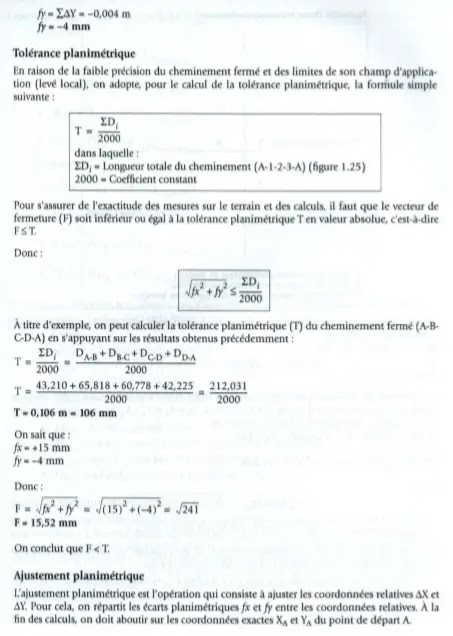For the calculation of the planimetric adjustments, the same method is used as that used in the case of the straight path (that is to say the method of proportional parallels).